# Generative Scribbling

##### Share:

Outline of Algorithm

The algorithm has 6 main sections:

1. Setup Function, scribble layers are generated here using p5’s createGraphic layers to avoid redrawing in the draw function. The setup function first determines the layers array and colors array, then it executes a loop that iterates the layers array and draw the scribbles inside the createGraphic layers
2. Draw Function, createGraphic layers are displayed here (along with the parallax effect optional feature). if parallax mode is activated, display the createGraphic layers with an offset determined by the cursor position. If not activated, each graphic layer will be displayed on top of each other with no offset.
3. Scribble Class, this class draws the individual scribbles, it is called in the setup function’s main loop.
4. Get Layer Data Functions, multiple functions that fetch the layer information for each variation (e.g. for Gorchov, Melita, Josef, etc.)
5. Boundary Checking Functions, these functions check if coordinate (x, y) lies inside a specific boundary
6. Utility Functions: pseudorandom number generator and random color generator.

Scribble Layer

Essentially, each piece is a superimposition of multiple scribble layers. Each layer has around 25–50 scribbles with varying amount of curve vertices. An example of a scribble layer in JS object notation looks something like this:

`scribbleLayer = { iterations: 300, boundary: [{type1, shape1}, {type2, shape2}, ...], closeness: 10, thicknessRange: 1.2, minThickness: 440, frame: [0.1, 0.9, 0.1, 0.9],}`

Each layer contains the following parameters:

• Iterations, number of scribbles per layer
• Boundaries, an array containing shape boundaries inside the frame, which could be in the form of circles, rectangles or random polygons
• Closeness, the minimum distance between the curve vertices (the closeness value is inversely proportional to the canvas length)
• Thickness Range, the range of how thick each scribble would be
• Minimum Thickness, the minimum width of a each scribble
• Frame, by default it is set to [0.1, 0.9, 0.1, 0.9]. The first two elements are the x-axis range (so the scribbles can only populate 10% to 90% of the canvas width), the last two elements are the y-axis range.

The hard part is to fine-tune these parameters so that the final output is not too chaotic, because I wanted the result to look similar to hand-drawn scribbles.

Drawing the Scribbles

Before drawing the scribbles, I determined the boundary mode (the layers in an array), colors array (I picked around 8–20 base colors), and color range array (the range of deviation from the base color — 4 values of range that change the hue, saturation, brightness and alpha from the base color). Then I iterate layers array in a for loop:

`Main Loop (Iterating through Layers) in the Setup Function----------------------------------------------------------1. Begin loop iterating through layer array a. Get random base color from colors array b. Begin another loop (iterations are determined by layer's iterations parameter) i. Draw scribble by calling the Scribble class, putting the layer information and random color from (a)2. Loop Ends`

The scribbles are drawn using P5’s curveVertex. To achieve this and for simplicity, I defined a class “scribble” with these constructor variables: closeness, line thickness, color, boundary, and number of vertices. Inside the class there is a display() function that draws the scribble. The pseudocode for one scribble is something like this:

`Display Function in Scribble Class----------------------------------1. Begin Shape2. Begin loop with number of points in the scribble a. Get random coordinate (x, y) b. Check if coordinate (x, y) is inside boundary i. If true, continue the loop, without including the coordinate ii. If false, add coordinate x and y as curve vertex3. End Shape`

Basically the scribbles are done in several nested loop. The range is somewhere between 50,000 to 200,000 total vertices.

Boundaries

There are three main boundaries: circles, partings and random polygon.

The circular boundary uses the common circle equation:

where (h, k) determines the position of the circle in the canvas, and r is the radius of the circle. I modified the equation above into a function to check if coordinate (x, y) lies inside the circle. The function will return true if it lies inside the circle, false otherwise:

`function inCircle (h, k, r, x, y){ let a = x - h; let b = y - k; return Math.pow(a, 2) + Math.pow(b, 2) <= Math.pow(r, 2)}`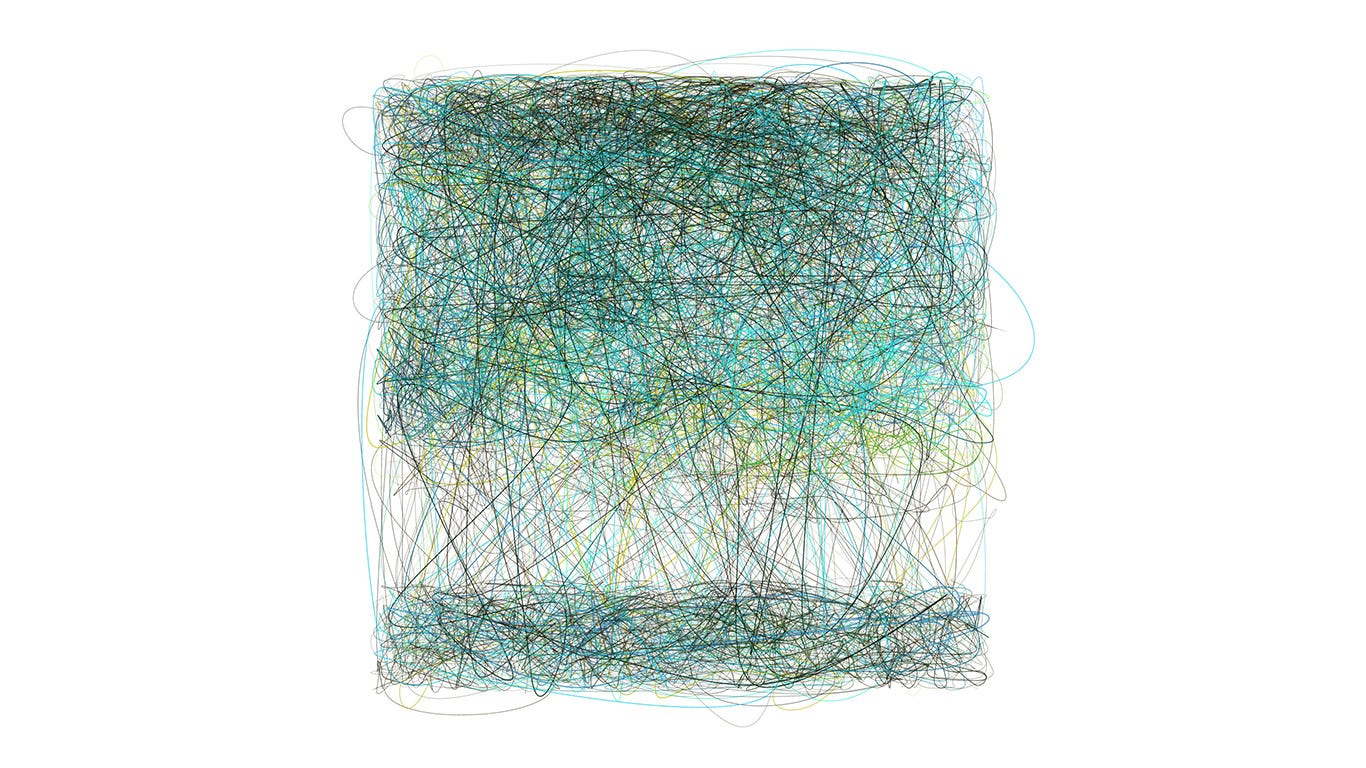Scribbled Boundaries #83 — an example that uses the parting boundary. As you can see, there is only 1 parting boundary on the lower part of the canvas

The parting boundary is a strip on the frame that is avoided, like in Sugimoto or Piet modes. The parameters of a parting boundary is the orientation of the strip (horizontal or vertical), width of the strip, and position of the strip. The function below check if coordinate (x, y) lies inside the strip — the cw variable is the canvas width. Where variables rx1, rx2 are lower and upper bounds of x values, while ry1, ry2 are the lower and upper bounds of y values.

`function inParting(parting, x, y){ if (parting.orientation == "vertical"){ let rx1 = cw*parting.location - cw*(parting.size/2) let rx2 = cw*parting.location + cw*(parting.size/2) if (x > rx1 && x < rx2) return true else return false } else { let ry1 = cw*parting.location - cw*(parting.size/2) let ry2 = cw*parting.location + cw*(parting.size/2) if (y > ry1 && y < ry2) return true else return false }}`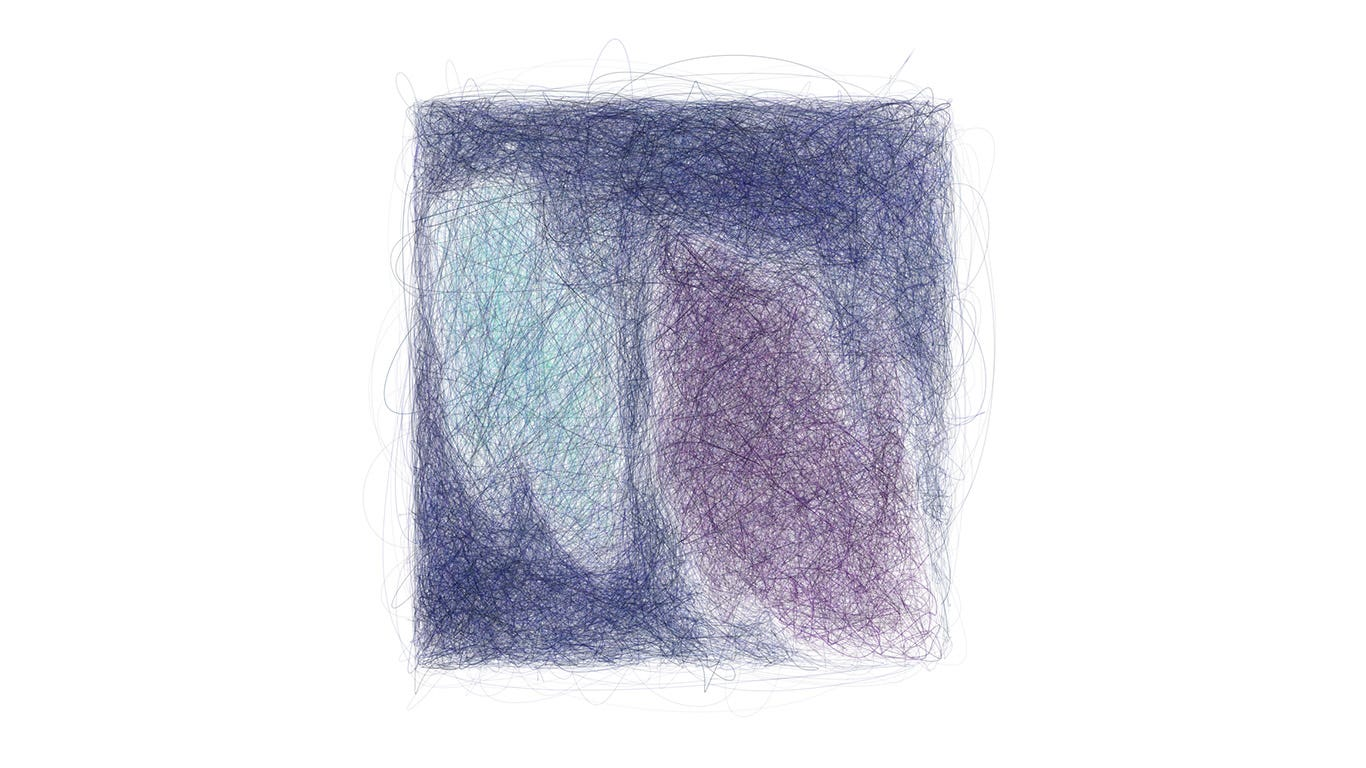Scribbled Boundaries #40 — two polygonal boundaries

Lastly, the polygon boundary uses a random n-sided polygon as the boundary. First, you generate n random coordinates that lies inside the frame. The coordinates need to be in the correct order or else it wouldn’t create a closed non-intersecting polygon. To do this, I used the following algorithm:

`1. Find left-most and right-most coordinates a and b2. Separate coordinates that lies above and below the line ab into two separate arrays A and B.3. Sort A in increasing order according to its x-values4. Sort B in decreasing order according to its x-values5. Merge arrays A and B.`

The above algorithm will create a closed polygon. But the next problem is to figure out to check if a coordinate (x, y) lies inside the polygon. This is referred to the point-in-polygon (PIP) problem in computational geometry. I implemented the ray-casting algorithm to check whether the point is inside the polygon. Essentially it checks the number of intersections a ray passed the polygon from the tested point (x, y) to (∞, y). If the number of passings is odd, the point lies inside the polygon, but if it is even, the point lies outside the polygon.

`count = 0 foreach side in polygon: if ray_intersects_segment(P,side) then count = count + 1 if is_odd(count) then return inside else return outside`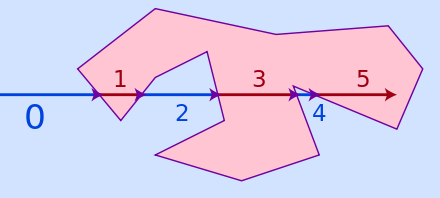Ray casting algorithm. The above illustration shows the ray that crosses the polygon.

Modes

The different modes use different ‘presets’ that mix-and-match different parameters together — including the different boundary types, frames, layers, and number of iterations. There are 14 modes in total; it would take forever for me to explain each mode, so I will pick and choose some of the outputs that I like, and kinda explain what’s going on in the mode.

#241 is in Josef mode, and as some of you might have guessed, it is an homage to Josef Albers’s Homage to the Square. This mode uses 4 nesting square of varying side lengths. This is achieved by using 4 layers: the outermost layer is the frame, with a square boundary inside of it of a smaller circle. The next layer has a smaller frame, with a smaller square boundary inside of it, and so on…

#358 is in Melita mode — it is also referred to as “split polygon” mode in the code. In this mode, the polygons are not intersecting/overlapping each other. This is achieved by first sectioning out the canvas into rectangular areas, and then define the polygons inside those areas. The number of layers depends on how many frames are there and how many polygons are there. So in this case, there are 3 layers, one for each polygon (the green and purple colored polygons), and one for the frame with the polygonal boundaries.

#469 is a Gorchov. In this mode, I have two parallel slits on the canvas (kinda reminds me of Thomas Young’s double-slit experiment). Just realized that it’s similar to the Rothko piece I attached earlier, this was purely by accident actually, I wasn’t inspired by that particular piece when came up with this mode.

The slits could sometimes collide and form one slit, like below:

I really like the simplicity and minimalism of Gorchovs. I can feel the calmness from it; almost like the light at the end of the tunnel. The chaos and irregularity subside if you look at it from afar.

#467 and #90 are in de Kooning mode. In this mode, I subdivide rectangular sections of the canvas and sometimes fills in a random polygons inside of it. This mode creates a wider range of outputs, which I really like. It’s hard to predict what will come out, and I love that aspect of this (you can see how different the two outputs above are).

#484 is in Twombly mode. In this mode I wanted to make a gradient, from more dense scribbles to less dense scribbles (also with an optional small polygon boundary in the middle). Again, I like the minimalism aspect of it, and it pays homage to Cy Twombly’s work too. This particular mode reminds me of the scribbles I drew when I was a child, I was just fascinated by the texture and colors of pencils and crayons. These scribbles represent a child’s innocence, wonder and curiosity.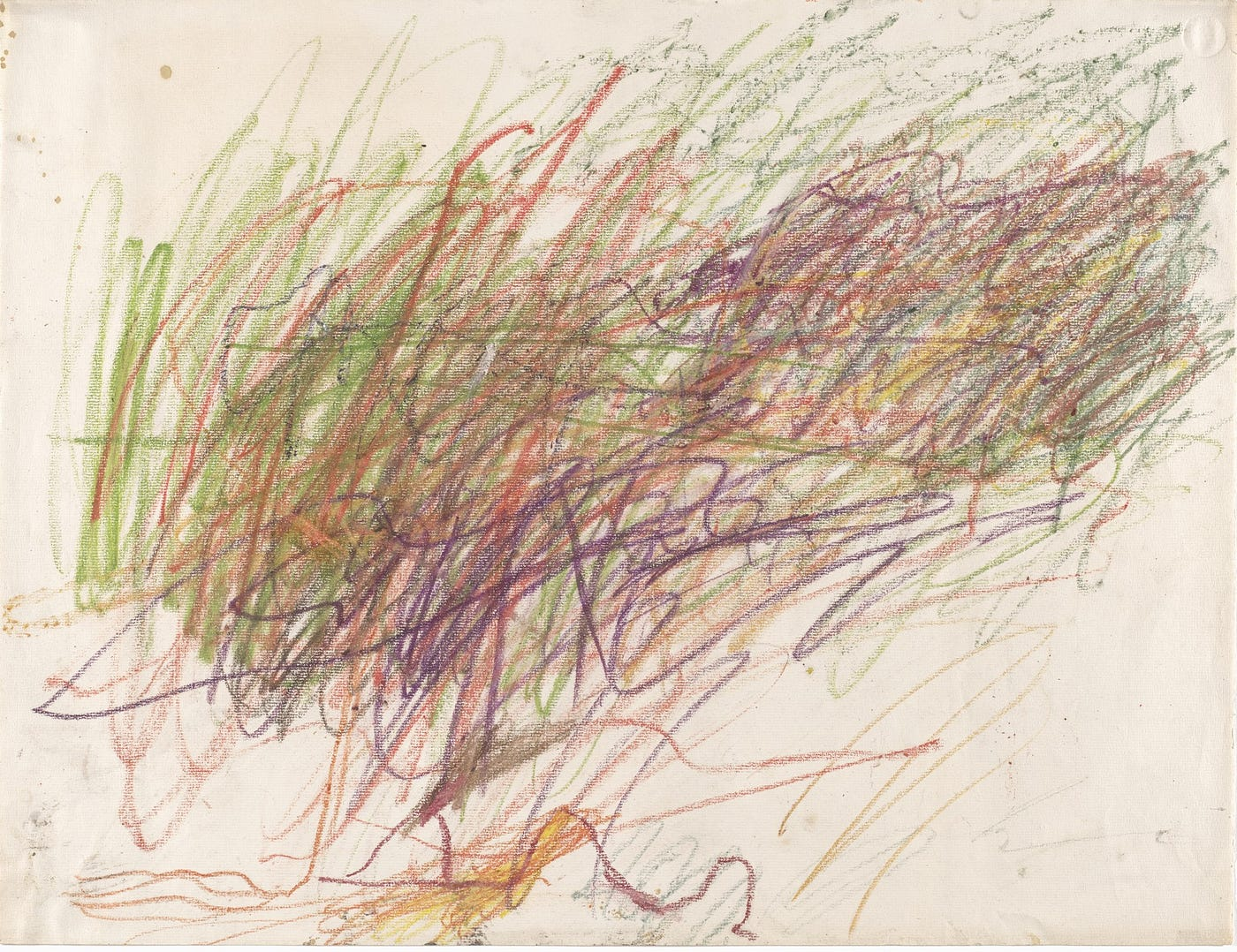Untitled (1954) by Cy Twombly — Gouache, crayon, and colored pencil on paper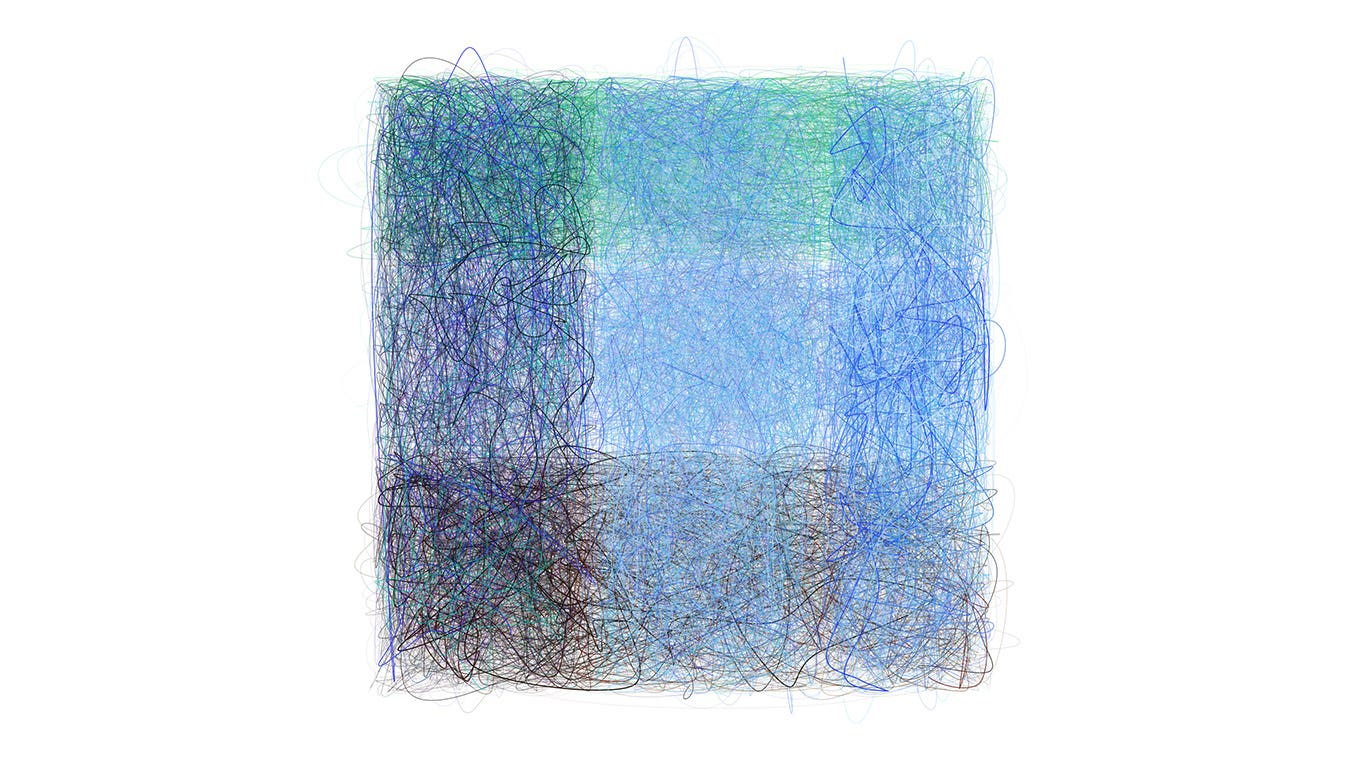Scribbled Boundaries #355

#355 is a Mark (an allusion to Mark Rothko). In original code, I named this mode “overlapping blocks.” As the name suggests, it fills different rectangular (vertical and horizontal) blocks with scribbles of random colors. I like how the blocks create new shades of color as it overlap each other.